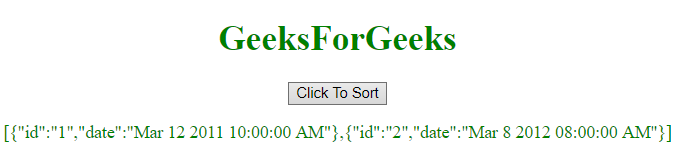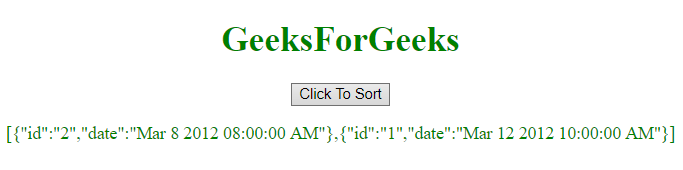# Sort an Object Array by Date in JavaScript

To sort an array of object by date, there is a number of methods but we’re going to see few of the most preferred methods.

Date Object: The Date object in JavaScript is used to represent a moment of time. This time value is since 1 January 1970 UTC (Coordinated Universal Time). We can create date using the Date object by calling the new Date() constructor as shown in the below syntax.
Syntax:

```new Date();
new Date(value);
new Date(dateString);
new Date(year, month, day, hours, minutes, seconds, milliseconds);
```

Parameters:

• value: This value is the number of milliseconds since January 1, 1970, 00:00:00 UTC.
• dateString: It represents a date format.
• year: It represents the integer values which ranges from years 1900 to 1999.
• month: It is represented by integer values which ranges from 0 for January to 11 for December.
• day: It is an optional parameter. It is represented by integer value for the day of the month.
• hours: It is optional parameter. It is represented by an integer value for the hour of the day.
• minutes: It is optional parameter. It is represented by an integer value for the minute of a time.
• seconds: It is optional parameter. It is represented by integer value for the second of a time.
• milliseconds: It is optional parameter. It is represented by integer value for the millisecond of a time.

Example 1: This example sorts the array of objects by date by using Date object.

 `  ` `<``html``>  ` `    ``<``head``>  ` `        ``<``title``>  ` `            ``JavaScript | Sort Object Array By Date ` `        ``  ` `    `` ` `     `  `    ``<``body` `style` `= ``"text-align:center;"``>  ` `         `  `        ``<``h1` `style` `= ``"color:green;"` `>  ` `            ``GeeksForGeeks  ` `        ``  ` `         `  `        ``<``button` `onclick` `= ``"geeks_outer()"``>  ` `            ``Click To Sort  ` `        ``  ` `     `  `        ``<``p` `id` `= ``"GFG_P"` `style` `= ``"color:green;"``> ` `         `  `        ``<``script``>  ` `            ``var array = [{id: "1", date: "Mar 11 2012 10:00:00 AM"}, ` `                        ``{id: "2", date: "Mar 8 2012 08:00:00 AM"}]; ` `             `  `            ``var el = document.getElementById("GFG_P"); ` `            ``function geeks_outer() { ` `                ``array.sort(function(a, b){ ` `     `  `                    ``return new Date(a.date) - new Date(b.date); ` `                ``}); ` `             `  `                ``el.innerHTML = JSON.stringify(array); ` `            ``} ` `             `  `        ``  ` `    ``  ` `                     `

Output:

• Before clicking on the button:• After clicking on the button:Example 2: This example is same as previous one but with a little modification in the sort function.

 `  ` `<``html``>  ` `    ``<``head``>  ` `        ``<``title``>  ` `            ``JavaScript | Sort Object Array By Date.  ` `        ``  ` `    `` ` `     `  `    ``<``body` `style` `= ``"text-align:center;"``>  ` `         `  `        ``<``h1` `style` `= ``"color:green;"` `>  ` `            ``GeeksForGeeks  ` `        ``  ` `         `  `        ``<``button` `onclick` `= ``"geeks_outer()"``>  ` `            ``Click To Sort ` `        ``  ` `         `  `        ``<``p` `id` `= ``"GFG_P"` `style` `= ``"color:green;"``> ` `         `  `        ``` `        ``<``script``>  ` `            ``var array = [{id: "1", date: "Mar 12 2012 10:00:00 AM"}, ` `                        ``{id: "2", date: "Mar 8 2012 08:00:00 AM"}]; ` `             `  `            ``var el = document.getElementById("GFG_P"); ` `         `  `            ``function geeks_outer() { ` `                ``array.sort(GFG_sortFunction); ` `                ``el.innerHTML = JSON.stringify(array); ` `            ``} ` `             `  `            ``function GFG_sortFunction(a, b) {  ` `                ``var dateA = new Date(a.date).getTime(); ` `                ``var dateB = new Date(b.date).getTime(); ` `                ``return dateA > dateB ? 1 : -1;  ` `            ``};  ` `        ``  ` `    ``  ` `                     `

Output:

• Before clicking on the button:• After clicking on the button:My Personal Notes arrow_drop_upCheck out this Author's contributed articles.

If you like GeeksforGeeks and would like to contribute, you can also write an article using contribute.geeksforgeeks.org or mail your article to contribute@geeksforgeeks.org. See your article appearing on the GeeksforGeeks main page and help other Geeks.

Please Improve this article if you find anything incorrect by clicking on the "Improve Article" button below.